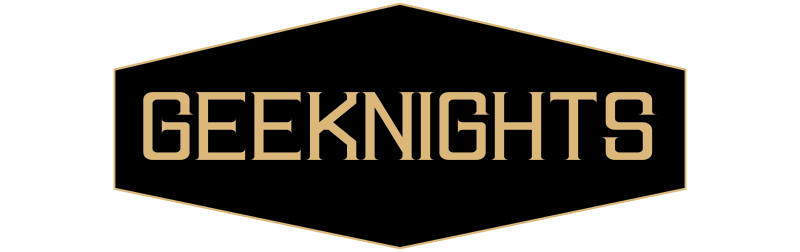# Math Puzzles

To start us off, here’s a modified version of something I saw last night:

A friend chooses a number from 1 to 16, then you ask 7 true/false questions to figure out what the number is. The catch is that the friend is allowed to lie on 1 question. You can only ask questions about the value of the number, not about meta things like “have you lied yet?” but otherwise can be as complicated as you want. You don’t necessarily need to find seven questions that work, just prove that it’s possible and give a general idea of how to find them. Bonus points for determining how many numbers you can distinguish if you’re allowed q questions with t lies.

There’s a branch of math that makes this problem pretty simple, but it should be solvable without it. I also came up with a second version I don’t have an answer for:

A friend chooses 1 of n numbers, then you ask q true/false questions and your friend lies on up to t of them. You can ask about the value of the number, whether the answer to a specific question was a lie, or any logical combination of these (ex: “Is it true that the number even AND you lied on question 3 OR the number is odd AND you told the truth on question 3”). For a given q and t, what’s the largest n for which you can always determine the chosen number?

So, in the first example, your friend will lie either 0 or 1 times?

Also, I don’t think there’s any additional value in being able to ask whether your friend has lied so far. Asking the same question again is equivalent to asking if the answer to that question was a lie.

Yeah, either 0 or 1 lie, although I’m pretty sure it isn’t any easier if they’re forced.

As for asking about whether they lied, I think it could be useful (with a very complex set of questions) but I can’t really explain why without going into my answer for the first part:

EDIT: nevermind, I’m pretty sure it doesn’t help

First Part Solution The problem can be treated as an noisy channel problem. The 7 questions map the chosen number to a 7 bit binary vector, with each bit corresponding to a "yes" or "no" answer. You then choose any bit and invert it (or don't) to represent the lie. The goal is to choose a mapping such that any two vectors can be distinguished for any noise.

This means you need every pair of vectors to have a Hamming distance of at least 3, which means that they differ in at least 3 places. If they vary in 2 places and one of those bits is flipped, there’s no way to tell which vector you started from. At distance 3 there’s always only a single possibility. The choice of questions is a solved problem: convert the number to binary and use Hamming (7, 4) code. There are many valid choices of questions though. For the general case, you can distinguish between n numbers if it’s possible to choose n binary vectors of length q such that every pair has a distance of at least 2t+1.

The reason asking about lies might be helpful is that instead of just mapping the number to a vector then adding the noise, you can make the mapping partly dependent on the noise, which may allow you to have some vectors at a distance less than 3. For example, you could have do vectors with a distance 2, but only when the lie occurred in an earlier question. Maybe. I’m really not sure what to make of it at this point.

EDIT: I thought about it some more, and I don’t think it can help, at least not in the case of a single lie. Right now the choice of the number and when to lie uniquely leads to a vectors of answers. The only way to solve for a larger pool of starting numbers is if for certain numbers, certain choices of when to lie led to the same answers for every question, which makes room for new number/lie combinations. But I’m pretty sure this is impossible.

I’ll probably rewrite that wall of text when I’m less tired.

First problem was kinda a bust, so here’s a better one: Prove that (a^5)b - a(b^5) is always a multiple of 10 when a and b are integers.